# corr

Model-implied temporal correlations of state-space model

## Syntax

``Cyy = corr(Mdl)``
``Cyy = corr(Mdl,Name,Value)``
``[Cyy,Cxx,Cyx] = corr(___)``
``[Cyy,Cxx,Cyx] = corr(___,'Params',estParams)``

## Description

The `corr` function returns model-implied temporal correlations and covariances of the state or measurement variables in a stationary, time-invariant state-space model. To determine whether the model captures characteristics present in the data, You can compare model-implied associations of present and lagged variables to sample analogues. Other state-space model tools to characterize the dynamics of a specified system include the following:

• The impulse response function (IRF), computed by `irf` and plotted by `irfplot`, traces the effects of a shock to a state disturbance on the measurement variables in the system.

• The forecast error variance decomposition (FEVD), computed by `fevd`, provides information about the relative importance of each state disturbance in affecting the forecast error variance of all measurement variables in the system.

### Fully Specified State-Space Model

example

``Cyy = corr(Mdl)` returns Corr(yt,yt – 1), the model-implied temporal correlation of each measurement variable of the fully specified, standard, stationary state-space model `Mdl`.`

example

``Cyy = corr(Mdl,Name,Value)` uses additional options specified by one or more name-value arguments. For example, `'Covariance',true,'NumLags',10` specifies returning temporal covariances Cov(yt,yt – h), h = 0 through 10.`

example

``[Cyy,Cxx,Cyx] = corr(___)` also returns Corr(xt,xt – h), the correlations between the state variables and their self-lags `Cxx`, and Corr(yt,xt – h), the correlations between the state variables and their self lags `Cxx` and the cross-correlations between the measurement variables and lags of the state variables `Cyx` using any of the input argument combinations in the previous syntaxes. h is the value of the `NumLags` name-value argument. `corr` returns covariances when the value of the `Covariance` name-value argument is `true`.`

### Partially Specified State-Space Model

example

``[Cyy,Cxx,Cyx] = corr(___,'Params',estParams)` uses the partially specified, standard state-space model `Mdl` and substitutes the parameter estimates `estParams` for all unknown parameters in the model.`

## Examples

collapse all

Explicitly create the state-space model

`$\begin{array}{l}{x}_{1,t}=0.9{x}_{1,t-1}+0.2{u}_{1,t}\\ {x}_{2,t}=0.1{x}_{1,t-1}+0.3{x}_{2,t-1}+{u}_{2,t}\\ {y}_{1,t}={x}_{1,t}\\ {y}_{2,t}={x}_{1,t}+{x}_{2,t}.\end{array}$`

```A = [0.9 0; 0.1 0.3]; B = [0.2 0; 0 1]; C = [1 0; 1 1]; Mdl = ssm(A,B,C,'StateType',[0 0])```
```Mdl = State-space model type: ssm State vector length: 2 Observation vector length: 2 State disturbance vector length: 2 Observation innovation vector length: 0 Sample size supported by model: Unlimited State variables: x1, x2,... State disturbances: u1, u2,... Observation series: y1, y2,... Observation innovations: e1, e2,... State equations: x1(t) = (0.90)x1(t-1) + (0.20)u1(t) x2(t) = (0.10)x1(t-1) + (0.30)x2(t-1) + u2(t) Observation equations: y1(t) = x1(t) y2(t) = x1(t) + x2(t) Initial state distribution: Initial state means x1 x2 0 0 Initial state covariance matrix x1 x2 x1 0.21 0.03 x2 0.03 1.10 State types x1 x2 Stationary Stationary ```

`Mdl` is an `ssm` model object. Because all parameters have known values, the object is fully specified.

Compute the temporal correlations of the measurement variables through lag 1.

`Cyy = corr(Mdl)`
```Cyy = Cyy(:,:,1) = 1.0000 0.4411 0.9000 0.4072 Cyy(:,:,2) = 0.4411 1.0000 0.3970 0.4212 ```

Rows correspond to lags, columns correspond to the latest observation of the measurement variable in the correlation, and pages correspond to the lagged measurement variable. For example,$\mathrm{Corr}\left({\mathit{y}}_{1,\mathit{t}}{\mathit{y}}_{2,\mathit{t}-1}\right)$ is `0.3970`.

Display a heatmap of the temporal correlations between the latest observation of each measurement variable and all lags of measurement variable 1.

```Corryy1 = Cyy(:,:,1); hm = heatmap(Corryy1); ylabel('h'); hm.YDisplayLabels = ["0" "1"]; xlabel('i') hm.XDisplayLabels = ["1" "2"]; title('Corr(y_{i,t},y_{1,t - h})')```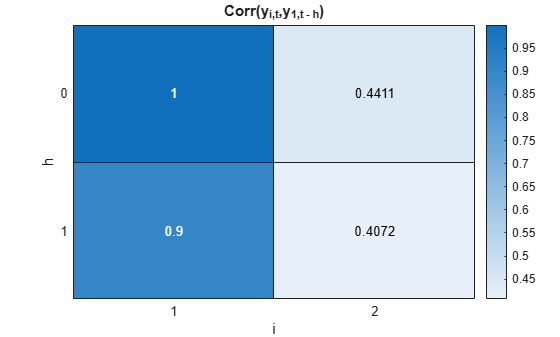Display a heatmap of the temporal correlations between the current observation of measurement variable 1 and all lags of all measurement variables.

```Corry1y = squeeze(Cyy(:,1,:)); hm = heatmap(Corry1y); ylabel('h'); hm.YDisplayLabels = ["0" "1"]; xlabel('j') hm.XDisplayLabels = ["1" "2"]; title('Corr(y_{1,t},y_{j,t - h})')```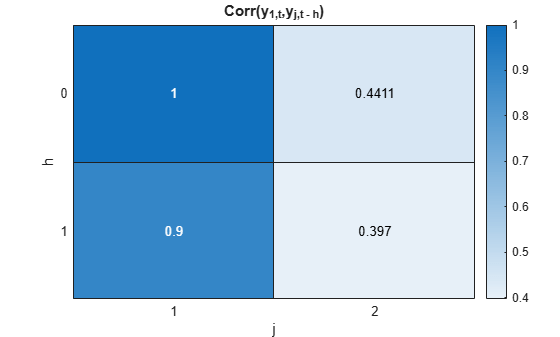Display a heatmap of the temporal correlations between the current observation of all measurement variables and the first lag of all measurement variables.

```Corryylag1 = squeeze(Cyy(2,:,:)); hm = heatmap(Corryylag1); ylabel('i'); hm.YDisplayLabels = ["1" "2"]; xlabel('j') hm.XDisplayLabels = ["1" "2"]; title('Corr(y_{i,t},y_{j,t - 1})')```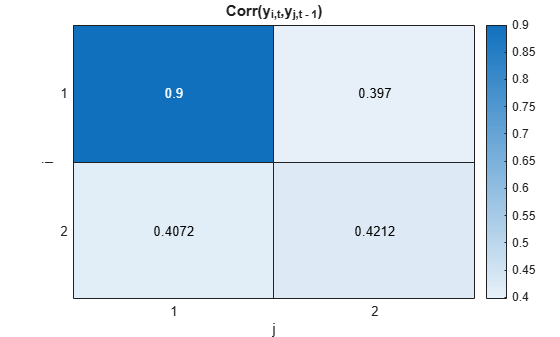Explicitly create the state-space model

`$\begin{array}{l}{x}_{1,t}=0.9{x}_{1,t-1}+0.2{u}_{1,t}\\ {x}_{2,t}=0.1{x}_{1,t-1}-0.3{x}_{2,t-1}+{u}_{2,t}\\ {y}_{1,t}={x}_{1,t}+{\epsilon }_{1,t}\\ {y}_{2,t}={x}_{1,t}+{x}_{2,t}+{\epsilon }_{2,t}.\end{array}$`

```A = [0.9 0; 0.1 -0.3]; B = [0.2 0; 0 1]; C = [1 0; 1 1]; D = eye(2); Mdl = ssm(A,B,C,D,'StateType',[0 0]);```

`Mdl` is an `ssm` model object.

Compute the temporal correlations of the measurement variables from lag 0 through 20.

```numlags = 20; Cyy = corr(Mdl,'NumLags',numlags);```

`Cyy` is a 21-by-2-by-2 array representing the 20-period temporal correlations of the measurement variables.

Display `Cyy(:,2,2)`, which is the model-implied autocorrelation of ${\mathit{y}}_{2,\mathit{t}}$.

`acfy2 = Cyy(:,2,2)`
```acfy2 = 21×1 1.0000 -0.0466 0.1267 0.0634 0.0723 0.0605 0.0558 0.0498 0.0449 0.0404 ⋮ ```

Generate a random path of measurements of length 200 from the model.

```rng(1); % For reproducibility Y = simulate(Mdl,200);```

Compute the sample autocorrelation function (ACF) of each variable for 2q0 lags.

```sacfy1 = autocorr(Y(:,1),'NumLags',numlags); sacfy2 = autocorr(Y(:,2),'NumLags',numlags);```

Visually compare the model-implied and sample ACF of each measurement variable.

```acfy1 = Cyy(:,1,1); plot([acfy1 sacfy1]) xticklabels(0:numlags) ylabel("Autocorrelation") xlabel("Lags") legend(["ACF(y_{1,t})" "Sample ACF(y_{1,t})"]) title("ACF(y_{1,t})") axis tight```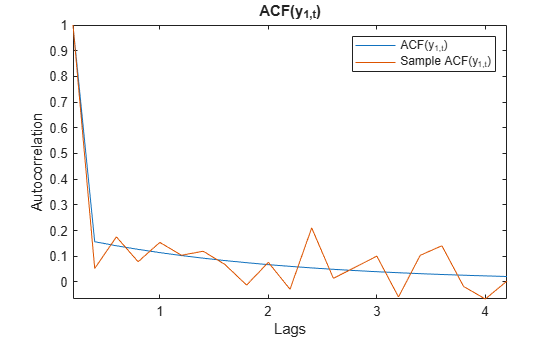```plot([acfy2 sacfy2]) xticklabels(0:numlags) ylabel("Autocorrelation") xlabel("Lags") legend(["ACF(y_{2,t})" "Sample ACF(y_{2,t})"]) title("ACF(y_{2,t})") axis tight```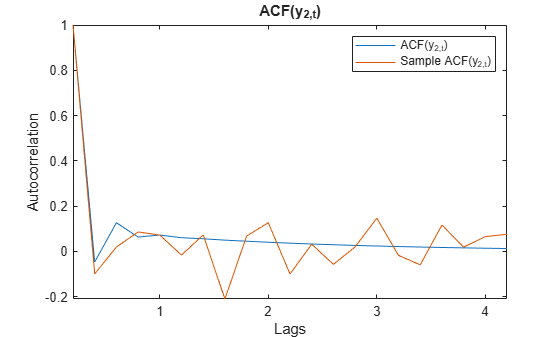Explicitly create the state-space model

`$\begin{array}{l}{x}_{1,t}=0.9{x}_{1,t-1}+0.2{u}_{1,t}\\ {x}_{2,t}=0.1{x}_{1,t-1}-0.3{x}_{2,t-1}+{u}_{2,t}\\ {y}_{1,t}={x}_{1,t}+{\epsilon }_{1,t}\\ {y}_{2,t}={x}_{1,t}+{x}_{2,t}+{\epsilon }_{2,t}.\end{array}$`

```A = [0.9 0; 0.1 -0.3]; B = [0.2 0; 0 1]; C = [1 0; 1 1]; D = eye(2); Mdl = ssm(A,B,C,D,'StateType',[0 0]);```

`Mdl` is an `ssm` model object.

Compute the temporal correlations of the measurement and state variables, as well as their cross-correlations.

`[Cyy,Cxx,Cyx] = corr(Mdl);`

Each output variable is a 2-by-2-by-2 array containing temporal correlations from lag 0 to 1. `Cyy` contains the correlations between the measurement variables, `Cxx` contains the correlations among the state variables, and `Cyx` contains cross-correlations between the current observation of the measurement variables and lagged state variables.

Plot a heatmap of the correlations between ${\mathit{x}}_{1,\mathit{t}}$ and the lags of all state variables.

```Cx1x = squeeze(Cxx(:,1,:)); hm = heatmap(Cx1x); ylabel('h'); hm.YDisplayLabels = ["0" "1"]; xlabel('j') hm.XDisplayLabels = ["1" "2"]; title('Corr(x_{1,t},x_{j,t - h})')```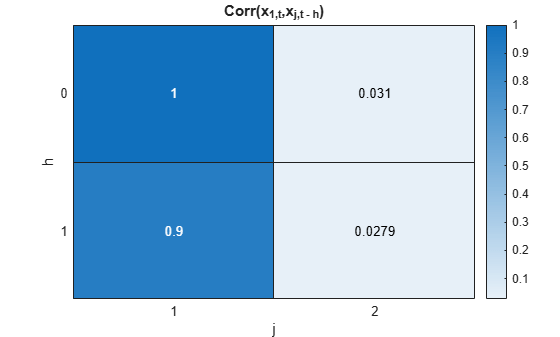Plot a heatmap of the cross-correlations between all measurement variables and the lags of ${\mathit{x}}_{2,\mathit{t}}$.

```Cyx2 = Cyx(:,:,2); hm = heatmap(Cyx2); ylabel('h'); hm.YDisplayLabels = ["0" "1"]; xlabel('i') hm.XDisplayLabels = ["1" "2"]; title('Corr(y_{i,t},x_{2,t - h})')```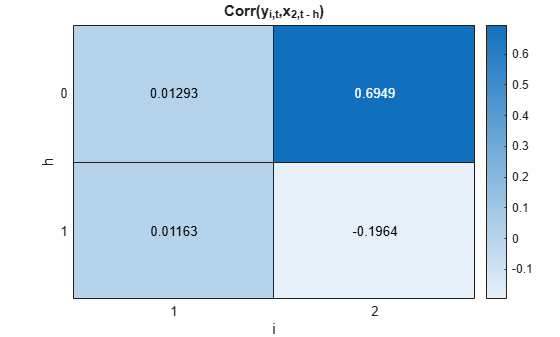Simulate data from a known model, fit a model to the data, and then compare sample and model-implied covariances.

Simulate Data

Explicitly create the state-space model

`$\begin{array}{l}{x}_{1,t}=0.9{x}_{1,t-1}+0.2{u}_{1,t}\\ {x}_{2,t}=0.1{x}_{1,t-1}-0.3{x}_{2,t-1}+{u}_{2,t}\\ {y}_{1,t}={x}_{1,t}+{\epsilon }_{1,t}\\ {y}_{2,t}={x}_{1,t}+{x}_{2,t}+{\epsilon }_{2,t}.\end{array}$`

```ADGP = [0.9 0; 0.1 -0.3]; BDGP = [0.2 0; 0 1]; CDGP = [1 0; 1 1]; DDGP = eye(2); DGP = ssm(ADGP,BDGP,CDGP,DDGP,'StateType',[0 0]);```

Generate a random path of measurements of length 500 from the model.

```rng(1); % For reproducibility numobs = 500; Y = simulate(DGP,numobs);```

Fit Model to Data

Create a state-space model template to fit to the data by replacing each nonzero state parameter of the data-generating process with a `NaN` value.

```A = [NaN 0; NaN NaN]; B = [NaN 0; 0 NaN]; Mdl = ssm(A,B,CDGP,DDGP,'StateType',[0 0]);```

Fit the model template to the data. Specify a random set of positive starting values. Return the vector of estimated parameters.

`[~,estParams] = estimate(Mdl,Y,abs(rand(5,1)));`
```Method: Maximum likelihood (fminunc) Sample size: 500 Logarithmic likelihood: -1694.08 Akaike info criterion: 3398.15 Bayesian info criterion: 3419.23 | Coeff Std Err t Stat Prob --------------------------------------------------- c(1) | 0.91506 0.04229 21.63954 0 c(2) | -0.25898 0.25406 -1.01933 0.30805 c(3) | -0.15383 0.08243 -1.86621 0.06201 c(4) | -0.16808 0.04926 -3.41221 0.00064 c(5) | 1.19275 0.06842 17.43153 0 | | Final State Std Dev t Stat Prob x(1) | -0.12293 0.30568 -0.40217 0.68756 x(2) | -0.80608 0.79263 -1.01697 0.30917 ```

Compute Covariances

Compute model-implied temporal covariances of the measurement variables by passing the state-space model template and estimated parameters to `corr`. Return the covariances instead of the correlations.

`Covyy = corr(Mdl,'Params',estParams,'Covariance',true)`
```Covyy = Covyy(:,:,1) = 1.1737 0.1376 0.1589 0.1195 Covyy(:,:,2) = 0.1376 2.5676 0.1259 -0.1297 ```

`Covyy` is a 2-by-2-by-2 array of temporal covariances of the measurement variables.

Compute the sample covariances of the measurement variables and their first lags.

```AugData = lagmatrix(Y,[0 1]); SCovyy = cov(AugData(2:end,:));```

Compare Covariances

Compare the model-implied temporal covariances and the sample covariances.

```names = ["y_1t" "y_2t" "y_1t-1" "y_2t-1"]; Covy1y = squeeze(Covyy(:,1,:))'; Covy2y = squeeze(Covyy(:,2,:))'; CovyLag1 = [Covy1y(:,2) Covy2y(:,2) Covy1y(:,1) Covy2y(:,1)]'; ModelCovariances = array2table([Covy1y(:) Covy2y(:) CovyLag1],'RowNames',names,... 'VariableNames',names)```
```ModelCovariances=4×4 table y_1t y_2t y_1t-1 y_2t-1 _______ ________ _______ ________ y_1t 1.1737 0.13759 0.15891 0.1259 y_2t 0.13759 2.5676 0.11949 -0.12972 y_1t-1 0.15891 0.11949 1.1737 0.13759 y_2t-1 0.1259 -0.12972 0.13759 2.5676 ```
`SampleCovariances = array2table(SCovyy,'RowNames',names,'VariableNames',names)`
```SampleCovariances=4×4 table y_1t y_2t y_1t-1 y_2t-1 ________ ________ ________ ________ y_1t 1.2459 0.22058 0.11689 0.070475 y_2t 0.22058 2.5332 0.074687 -0.17466 y_1t-1 0.11689 0.074687 1.2437 0.22158 y_2t-1 0.070475 -0.17466 0.22158 2.5419 ```

The model-implied and sample covariances appear to be similar in magnitude. Note that the covariances are invariant to the reference time; for example, Cov(${\mathit{y}}_{1,\mathit{t}},{\mathit{y}}_{2,\mathit{t}}$) = Cov(${\mathit{y}}_{1,\mathit{t}-1},{\mathit{y}}_{2,\mathit{t}-1}$).

## Input Arguments

collapse all

Standard, stationary state-space model, specified as an `ssm` model object returned by `ssm` or its `estimate` function.

• Temporal moments are well defined for stationary states. Therefore, `corr` issues an error if one or more of the following conditions apply:

• At least one state is nonstationary (`Mdl.StateType` contains at least one value of `2`).

• At least one coefficient is time varying.

• Either the measurement or state variable is dimension varying.

• If `Mdl` is partially specified (that is, it contains unknown parameters), specify estimates of the unknown parameters by using the `'Params'` name-value argument. Otherwise, `corr` issues an error.

• The initial covariance matrix `Mdl.Cov0` is implied by the transition equation. Therefore, `corr` ignores `Mdl.Cov0` and values corresponding to `Cov0` in the value of `Params`.

### Name-Value Arguments

Specify optional comma-separated pairs of `Name,Value` arguments. `Name` is the argument name and `Value` is the corresponding value. `Name` must appear inside quotes. You can specify several name and value pair arguments in any order as `Name1,Value1,...,NameN,ValueN`.

Example: `'Covariance',true,'NumLags',10` specifies returning Cov(yt,yt – 10), covariances of self- and cross-lags of the measurement variables from lags 0 through 10.

Maximum number of state or measurement variable lags to include in the computation, specified as a nonnegative integer. `corr` returns associations from lags 0 through `NumLags`.

Example: `'NumLags',10`

Data Types: `double`

Estimates of the unknown parameters in the partially specified state-space model `Mdl`, specified as a numeric vector.

If `Mdl` is partially specified (contains unknown parameters specified by `NaN`s), you must specify `Params`. The `estimate` function returns parameter estimates of `Mdl` in the appropriate form. However, you can supply custom estimates by arranging the elements of `Params` as follows:

• If `Mdl` is an explicitly created model (`Mdl.ParamMap` is empty `[]`), arrange the elements of `Params` to correspond to hits of a column-wise search of `NaN`s in the state-space model coefficient matrices, initial state mean vector, and covariance matrix.

• If `Mdl` is time invariant, the order is `A`, `B`, `C`, `D`, `Mean0`, and `Cov0`.

• If `Mdl` is time varying, the order is `A{1}` through `A{end}`, `B{1}` through `B{end}`, `C{1}` through `C{end}`, `D{1}` through `D{end}`, `Mean0`, and `Cov0`.

• If `Mdl` is an implicitly created model (`Mdl.ParamMap` is a function handle), the first input argument of the parameter-to-matrix mapping function determines the order of the elements of `Params`.

If `Mdl` is fully specified, `corr` ignores `Params`.

Example: Consider the state-space model `Mdl` with `A = B = [NaN 0; 0 NaN]` , `C = [1; 1]`, `D = 0`, and initial state means of 0 with covariance `eye(2)`. `Mdl` is partially specified and explicitly created. Because the model parameters contain a total of four `NaN`s, `Params` must be a 4-by-1 vector, where `Params(1)` is the estimate of `A(1,1)`, `Params(2)` is the estimate of `A(2,2)`, `Params(3)` is the estimate of `B(1,1)`, and `Params(4)` is the estimate of `B(2,2)`.

Data Types: `double`

Flag for returning the temporal covariances instead of the correlations, specified as a value in this table.

ValueDescription
`false`Output arguments represent temporal correlations
`true`Output arguments represent temporal covariances

Example: `'Covariance',true`

Data Types: `logical`

## Output Arguments

collapse all

Temporal associations of the measurement variables (correlations or covariances), returned as a (`NumLags` + 1)-by-n-by-n numeric array.

`Cyy(h + 1,i,j)` is the temporal association between y`i`,t and y`j`,th, for `h` = 0,1,2,...,`NumLags`, `i` = 1,2,...,n (number of measurement variables), and `j` = 1,2,...,n.

Temporal associations of the state variables, returned as a (`NumLags` + 1)-by-m-by-m numeric array.

`Cxx(h + 1,i,j)` is the temporal association between x`i`,t and x`j`,th, for `h` = 0,1,2,...,`NumLags`, `i` = 1,2,...,m (number of state variables), and `j` = 1,2,...,m.

Temporal cross-associations between measurement and state variables, returned as a (`NumLags` + 1)-by-n-by-m numeric array.

`Cyx(h + 1,i,j)` is the temporal association between y`i`,t and x`j`,th, for `h` = 0,1,2,...,`NumLags`, `i` = 1,2,...,n, and `j` = 1,2,...,m.

collapse all

### Model-Implied Temporal Associations

Model-implied temporal correlations and covariances measure self- and cross-lag associations between measurement and state variables in a state-space model, as prescribed by the model. To facilitate model specification, you can compare model-implied temporal correlations and covariances to sample analogues.

Consider the time-invariant state-space model at time t

`$\begin{array}{l}{x}_{t}=A{x}_{t-1}+B{u}_{t}\\ {y}_{t}=C{x}_{t}+D{\epsilon }_{t}.\end{array}$`

Consider a demeaned state-space model represented by state and measurement variables ${\stackrel{˜}{x}}_{0,t}$ and ${\stackrel{˜}{y}}_{0,t}$:

1. Append the state-space model with an appropriately sized constant state vector representing an intercept.

`$\begin{array}{l}\left[\begin{array}{c}{x}_{0,t}\\ 1\end{array}\right]=\left[\begin{array}{cc}{A}_{0}& {A}_{1}\\ 0& 1\end{array}\right]\left[\begin{array}{c}{x}_{0,t-1}\\ 1\end{array}\right]+\left[\begin{array}{c}B\\ 0\end{array}\right]{u}_{t}\\ {y}_{t}=\left[\begin{array}{cc}{C}_{0}& {C}_{1}\end{array}\right]\left[\begin{array}{c}{x}_{0,t}\\ 1\end{array}\right]+D{\epsilon }_{t}.\end{array}$`

2. Demean the variables.

`$\begin{array}{l}{\stackrel{˜}{x}}_{0,t}={x}_{0,t}-E\left({x}_{0,t}\right)={x}_{0,t}-{\left(I-{A}_{0}\right)}^{-1}{A}_{1}\\ {\stackrel{˜}{y}}_{0,t}={y}_{0,t}-E\left({y}_{0,t}\right)={y}_{0,t}-{C}_{1}-{C}_{0}{\left(I-{A}_{0}\right)}^{-1}{A}_{1}.\end{array}$`

3. Demean the state-space model and drop constant terms that do not affect the covariance.

`$\begin{array}{l}{\stackrel{˜}{x}}_{0,t}={A}_{0}{\stackrel{˜}{x}}_{0,t-1}+B{u}_{t}\\ {\stackrel{˜}{y}}_{0,t}={C}_{0}{\stackrel{˜}{x}}_{0,t}+D{\epsilon }_{t}.\end{array}$`

Because the difference between the full state-space model and the demeaned model is the inclusion of constant states, $\text{Cov}\left({x}_{t},{x}_{t-h}\right)=\text{Cov}\left({\stackrel{˜}{x}}_{0,t},{\stackrel{˜}{x}}_{0,t-h}\right)={\Gamma }_{0,h},$ which implies

`$Cxx\left(1,:,:\right)={\Gamma }_{0,0}={A}_{0}{\Gamma }_{0,0}{A}_{0}\prime +BB\prime .$`

Let ${\stackrel{˜}{\Gamma }}_{0,0}$ be the solution to the equation. Using the demeaned state equation,

`$Cxx\left(h+1,:,:\right)={\Gamma }_{0,h}={A}_{o}{\Gamma }_{h-1};h=1,2,....$`

The preceding results imply the following:

• $Cyy\left(1,:,:\right)=\text{Cov}\left({y}_{t},{y}_{t}\right)={C}_{0}{\Gamma }_{0,0}{C}_{0}^{\prime }+D{D}^{\prime }.$

• $Cyy\left(h+1,:,:\right)=\text{Cov}\left({y}_{t},{y}_{t-h}\right)={C}_{0}{\Gamma }_{0,h}{C}_{0}^{\prime };h=1,2,....$

• $Cyx\left(h+1:,:\right)=\text{Cov}\left({y}_{t},{x}_{t-h}\right)={C}_{0}{\Gamma }_{0,h};h=0,1,2....$

## Tips

• To obtain an association matrix of lead variables from an association matrix of lagged variables, use the identity

`$C\left({a}_{t},{b}_{t+h}\right)=C{\left({a}_{t},{b}_{t-h}\right)}^{\prime },$`

where:

• C is an association function, either Corr or Cov.

• at and bt are yt or xt.

### Topics

Introduced in R2021a